# Analyze and Simulate Control Systems in Wolfram|Alpha

January 4, 2012 —Comments Off

What do your alarm clock, thermostat, coffeemaker, car radio, anti-lock brakes—and almost every other electrical and mechanical device you encounter in your daily life—all have in common? They are all examples of “control systems,” one of the most ubiquitous yet unseen modern technologies. A control system is any system or device that controls or regulates the behavior of another system. Using various kinds of sensors and actuators, these systems automatically control most common appliances, industrial processes, and even your body’s own biological processes!

Take your home’s humble thermostat. The temperature of your home depends on many factors, especially how long and how recently the home’s furnace was on. With a thermostat installed, the reverse is also true: the state of the furnace depends on the temperature of the house (it comes on if the temperature is too low, and turns off if the temperature is too high). There is a closed loop of causation formed between the home’s temperature and the state of the furnace. By design, the thermostat creates a kind of closed loop called a “negative feedback loop,” which tends to stabilize the temperature around a desired value. Most control systems are like this: sensors feed information back into the system, which is then used to decide on an action.

In Version 8 of Mathematica, we added lots of functions that analyze, design, and simulate a variety of systems, including control systems. We have now incorporated these capabilities into Wolfram|Alpha with the ability to understand some common systems by name.

A simple example is the integrator. An integrator is a system that accumulates the values of some input over time. For example, the amount of gas your car has used since you last filled up is basically an integral of the position of the gas pedal over that time. Put another way, gas is consumed at a rate proportional to how far the gas pedal is depressed. Typing “control system: integrator” into Wolfram|Alpha gives a list of properties, graphs, and an interactive response plot where you can see what happens to the output for several common inputs: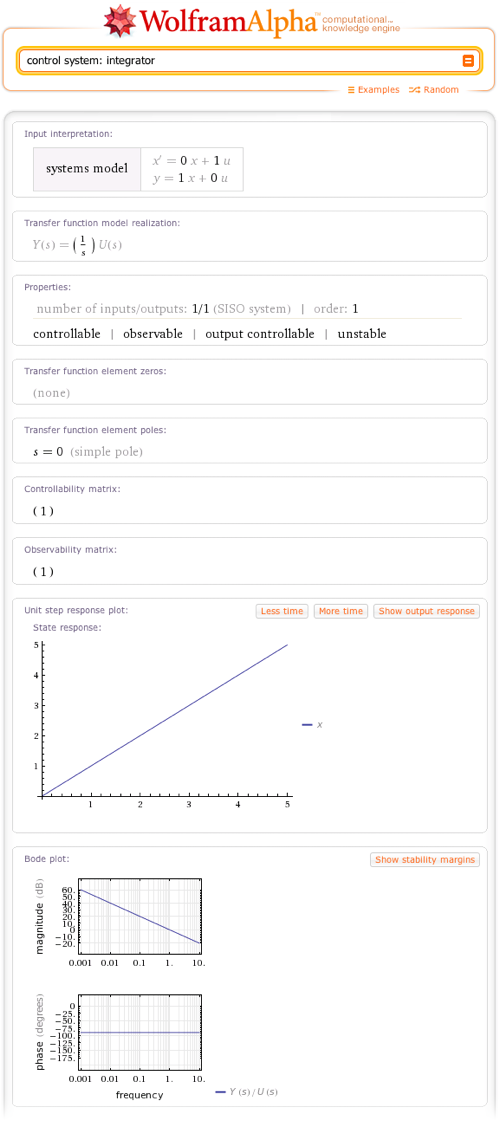Another standard type of control system Wolfram|Alpha knows about is the PID controller. Usually in the form of a programmable microchip, a PID controller can electronically control something based on the value (or a proportion of the value), the total accumulation (integral), and the rate of change (derivative) of some quantity. Type “PID controller,” and Wolfram|Alpha gives you a custom “calculator” that allows you to vary the strength of the proportional, integral, and derivative “gains” and shows how these variations affect the properties of the controller: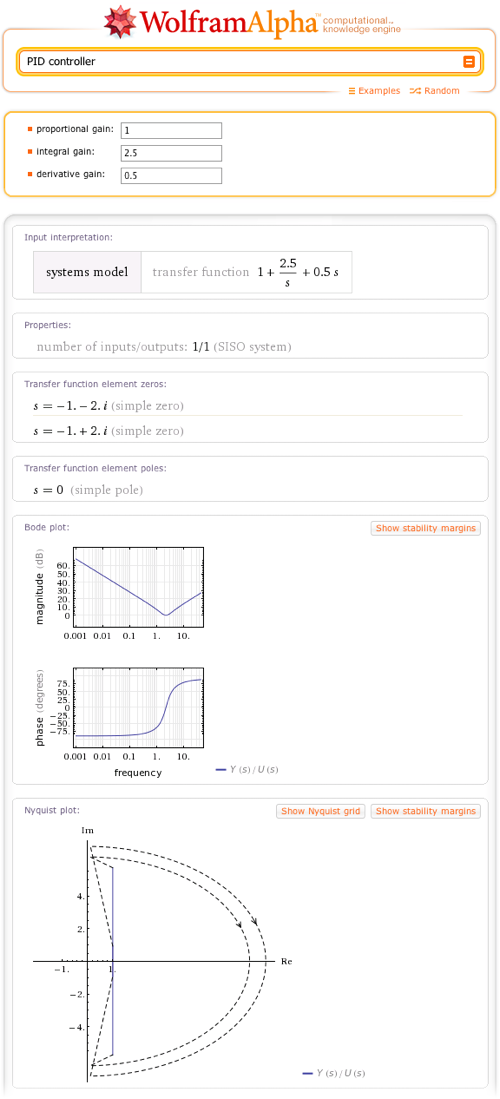In addition to this repertoire of standard control systems, which we will be expanding in the near future, Wolfram|Alpha can also understand and analyze custom systems, specified using either state space or transfer function models. These models provide a general framework to mathematically describe the input-output behavior of systems and are commonly used to model a complex process, whether a natural phenomenon, a control system, or something else. Wolfram|Alpha is equipped to handle both continuous- and discrete-time systems modeled using this framework.

In a state space model representation, variables are related to each other using ordinary differential equations. Some of these variables are the “input,” others are the “output,” and some are just internal to the system, called “state” variables. Wolfram|Alpha uses the standard convention, in which input variables are denoted by u, output variables by y, and state variables by x. As of this writing, Wolfram|Alpha can only analyze linear state space models, which are expressible as a system of vector differential equations:

x′(t) = Ax(t) + Bu(t)
y(t) = Cx(t) + Du(t)

…where A, B, C, and D are matrices of complex numbers. For example, “A: {{1,0},{1,-1}} B: {{0.2},{1}} C: {{-1.3,1}} : response to the input u[t] = sin(8t)” shows what the state vector x does when this system is fed the input sin(8t):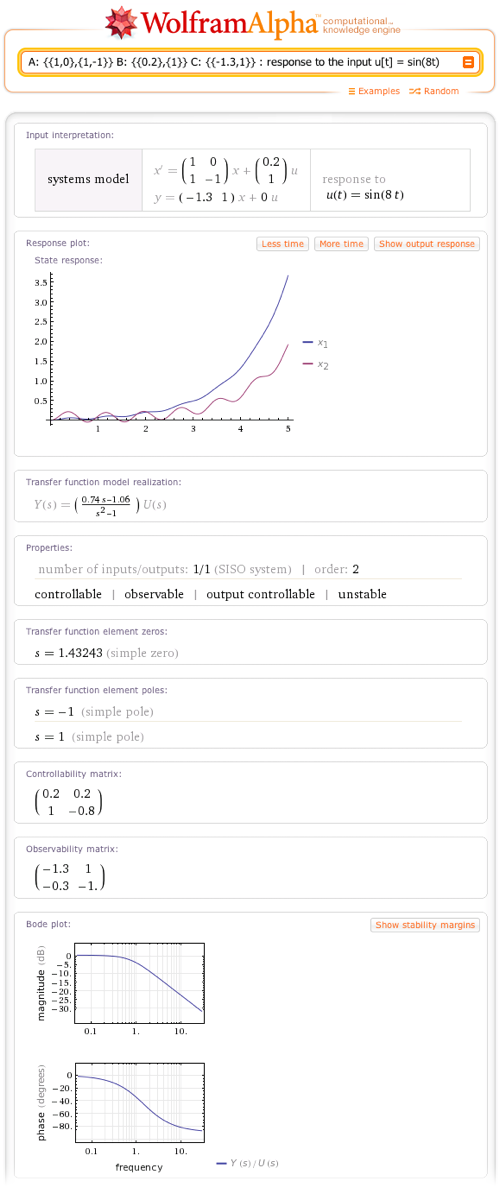The other way to represent a control system is with a transfer function. A transfer function is a ratio of U(s), the Laplace transform of the input u(t), over Y(s), the Laplace transform of the output y(t). In general, a transfer function is an n-by-m matrix of functions, where n is the number of outputs and m is the number of inputs.

Using either transfer function or state space inputs, you can specify a discrete model by entering a nonzero sampling period, such as 0.2: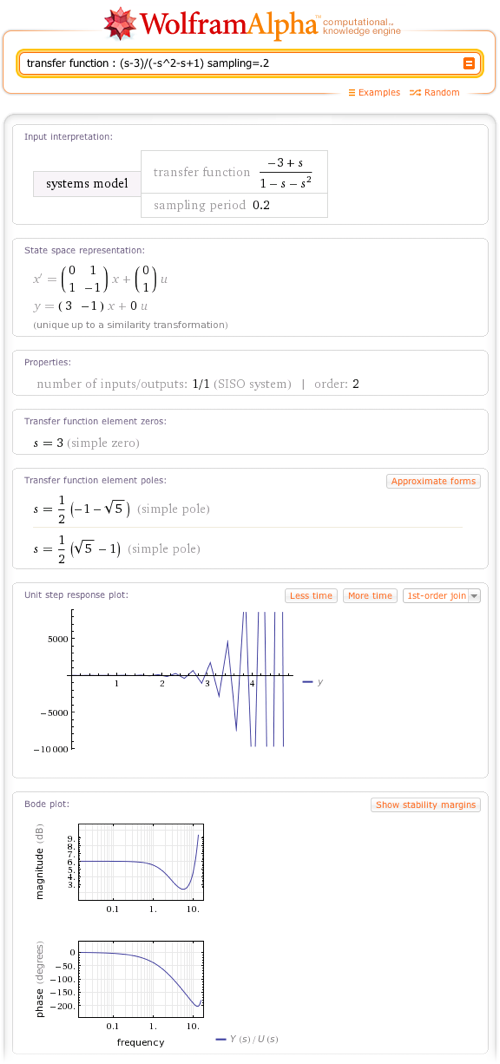You can also ask about specific properties or groups of properties. For example: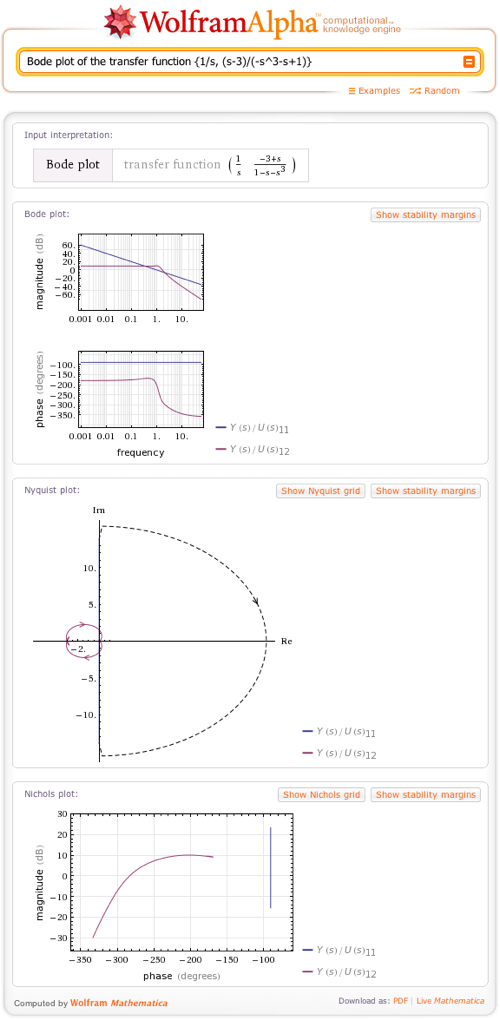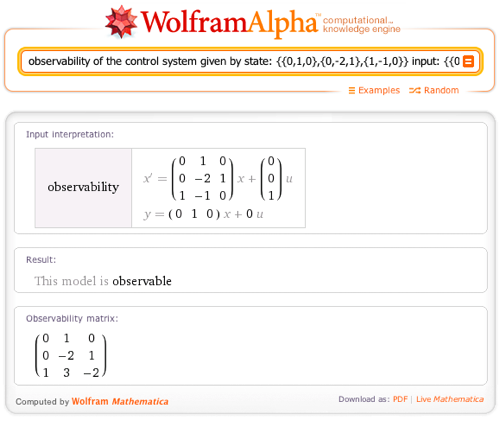Experiment by creating your own control systems or just play around with a standard system like the PID controller. You now have all the tools you need to analyze and test them. Whether you are a engineering student or someone trying to solve the world’s pressing problems, we hope this new feature will make controlling things a little easier.

To help people understand the big picture I suggest you make the point that a Control System belongs in Wolfram Alpha as a. formj of Algorithm. It therefore fits the very foundation of the spec that Wolfram Alpha as a computational Knowledge engine. Knowledge includes knowledge of algorithms not just isolated bits of data.

When curation gets into its stride all of WAs data will be linked by one giant algorithm. This will arise through WA being kept consistent as each new piece of data is added.

BG Volunteer Curator

Posted by Brian Gilbert January 10, 2012 at 3:45 am

Yes it’s good. How to you close the loop though? When you input a system and do the step response it does the open-loop response only. I assume there is a command for closed loop with unity negative feedback is there?

Posted by Tom May 21, 2012 at 4:31 pm

Thank you for your comment! The function you need is SystemsModelFeedbackConnect but unfortunately Wolfram|Alpha does not yet have this feature; Wolfram Desktop, however, does.

Posted by The Wolfram|Alpha Team May 2, 2014 at 9:07 am

Hi,

Any plans to release this to the mobile version?

Posted by Ant October 23, 2014 at 8:42 am

x(t)=10+20cos(2?(100)t+?/4)+10cos(2?(250)t)

Posted by Abdul Mannan October 27, 2014 at 11:06 am

I have figured out that this combo works now :

ToDiscreteTimeModel [ TransferFunctionModel [{{3(1+s)/(0.1+s) }},s] ,1,z ]

but I have yet to figure out how to get the (syntax?) subsequent Bode plot of it …an example would be useful (if it’s possible). If I could, I would have most everything I need in my work

Posted by Bill January 22, 2015 at 4:30 pm

If you need closed-loop with negative feedback, just divide your transfer function by 1+ this transfer function:
http://en.wikipedia.org/wiki/Closed-loop_transfer_function

Posted by Dawid February 25, 2015 at 2:05 am